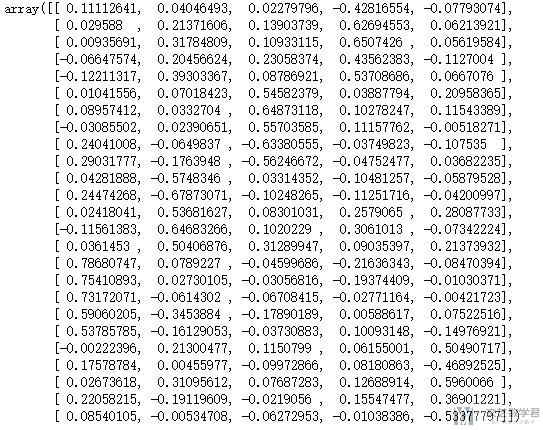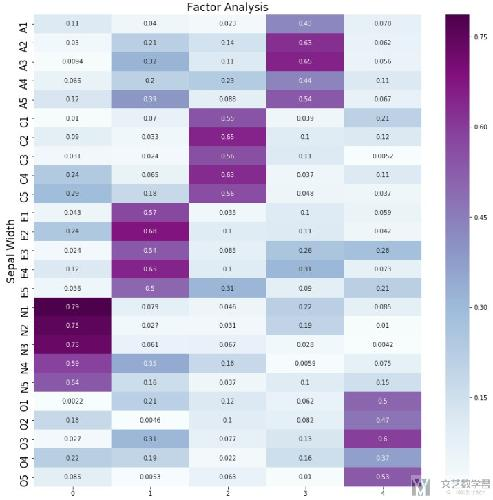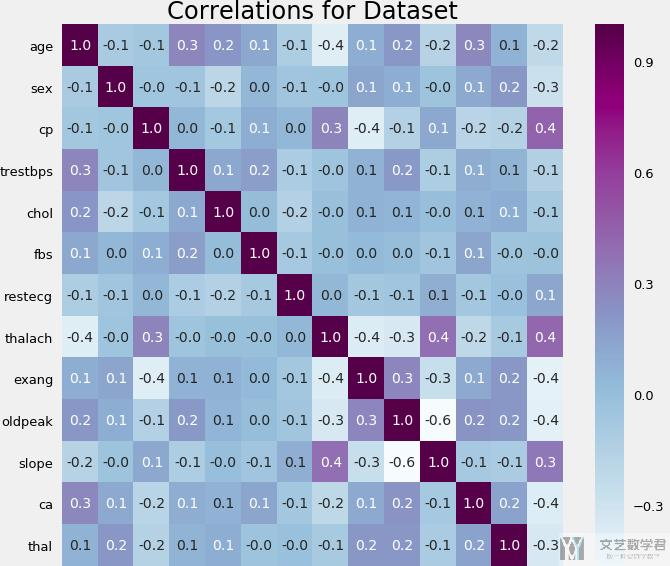# Seaborn绘图优化–矩阵可视化

• A+

## 一个具体的例子2. plt.figure(figsize = (14,14))
3. ax = sns.heatmap(df_cm, annot=True, cmap="BuPu")
4. # 设置y轴的字体的大小
5. ax.yaxis.set_tick_params(labelsize=15)
6. plt.title('Factor Analysis', fontsize='xx-large')
7. # Set y-axis label
8. plt.ylabel('Sepal Width', fontsize='xx-large')
9. plt.savefig('factorAnalysis.png', dpi=500)### ticks进行旋转

1. plt.yticks(rotation=0)

1. # 绘制相关系数矩阵
2. plt.figure(figsize = (10,10))
3. ax = sns.heatmap(data.corr(), annot=True, fmt='.1f', cmap="BuPu") # fmt表示保留的小数点
4. # 设置y轴的字体的大小
5. plt.yticks(rotation=0) # 让y轴的字进行旋转
6. # ax.yaxis.set_tick_params(labelsize=15)
7. plt.title('Correlations for Dataset', fontsize='xx-large')• 微信公众号
• 关注微信公众号
•• QQ群
• 我们的QQ群号
•